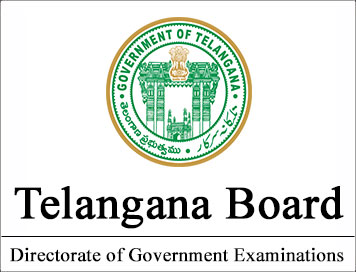NEW!  CBSE Papers PDF: Class-X, Class-XII

# Telangana State Board CLASS-12 Model Question Papers - Physics Paper II

Disclaimer: This website is not at associated with CBSE, For official website of CBSE visit - www.cbse.nic.in

##### Year : 2013-14

BOARD OF INTERMEDIATE EDUCATION, A.P, HYDERABAD
MODEL QUESTION
PAPER- PHYSICS II YEAR (W.E.F.2013-14)
Time: 3 Hours
Max.marks:60
SECTION-A

Answer all the questions (Very Short Answer Type) 10 X 2 =20

1. Write the formulae for the speed of sound in solids and gases.

2. What happens to the force between two charges if the distance between them is
(a) halved
(b) doubled.

3. Write the expression for electric intensity due to an infinite plane sheet of charge.

4. Write the expression for the Torque experienced a dipole placed in an external magnetic field.

5. On what factors does the resistance of a conductor depends ?

6. Number of turns in a coil are 100. When a current of 5A is flowing through the coil the magnetic
flux is 1-6 Wb. Find the self induction of the coil.

7. State Ampere’s law and Biot – Savart law.

8. The half life of 58Co is 72 days. Calculate its average life.

9. Draw the circuit symbols of P-N-P and N-P-N transistors.

10. Define modulation. Why is it necessary ?
SECTION-B

11. Define Doppler effect. Derive the expression for apparent frequency when the source is in
motion and observer is at rest.

12. Explain polarization by refraction.

13. Derive an expression for magnetic field induction on the equatorial line of a bar magnet.

14. Derive an expression for the equivalent capacity when capacitors are connected in series.

15. The balance point in metre bridge experiment is obtained at 30 cm from the left. If the right gap
contains 3.5 W, what is the resistance in the left gap?

16. Derive the balancing condition of a Wheatstone bridge.

17. Explain the working of a Nuclear Reactor with the help of a labelled diagram.

18. Describe how a Semi-Conductor diode is used as a half wave rectifier.

SECTION-C

19. Describe the construction and working of a compound microscope with a neat diagram.
Derive the expression for its magnification.

20. Describe the construction and working of a moving coil galvanometer. Obtain the relation
between current and deflection of the coil.

The resistance of M.C.G is 5W. The maximum current it can measure is 0.015A. How would
you convert it into voltmeter to measure 1.5 volts?

21. State Bohr’s Postulates. Derive the expression for the radius of the first orbit in a Hydrogen atom.
Radius of the first orbit of a Hydrogen atom is 5.3 x 10-11 m.
What are the radii of the n2 and n3 orbits?

*****